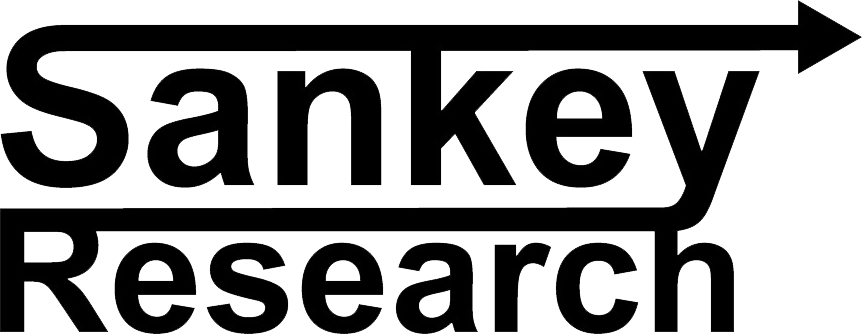• cl72.37-0.14%
• ng2.33+0.13%
• xom120.21+3.2643%
• rwe39.02-0.61%
• eolu-b201.1-1.61%
• cvx171.08+1.9547%
• cop123.09+2.9697%
• shel58.2+0.87%
• bp39.48+2.1739%
• eog129.81+2.3093%
• pxd234.09+2.7296%
• oxy65.6+3.8797%
• ovv48.91+5.0698%
• fang157.23+2.7244%
• mro27.39+4.1841%
• vlo143.95+1.674%
• psx120.8+1.1556%
• mpc152.43+0.9337%
• hfcNA+NA%
• dk29.28+1.4553%
• pbf54.26+1.9925%
• ar22.22+5.91%
• co76.74-0.04%
• apa42.38+3.24%
• dvn48.84+4.0256%
• mur46.42+2.6537%
• cnq66.02+2.436%
• su35.13+2.8095%
• swn6.38+3.2362%
• rrc32.13+4.0816%
• nog40.94+3.6981%
• eqt39.61+1.6684%
• slb60.8+2.8243%
• hal42.03+3.04%
• epd27.37+0.588%
• paa15.27+0.5267%
• et13.95+0.6494%
• kmi16.66+1.1536%
• lng165.23+1.9875%
• mmpNA+NA%
• enb33.63-0.1781%
• hes158.04+3.9463%
• eqnr33.04+3.0889%
• e32.5+1.849%
• repyy16.43+1.1077%
• cve20.98+3.0958%
• clrNA+NA%
• rig8.56+1.6627%
• d45.46-1.3026%
• elcpf4.280%
• esocf6.280%
• es58.77-1.5743%
• nee59.96-8.2339%
• dogef54.7+0.4813%
• ssezf20.14-2.1762%
• alb162.63-0.6233%
• apd285.26-0.5023%
• lthm17.09-1.4418%
• sqm54.84-1.3846%
• lpx55.01-0.1271%
• wy31.03+0.0322%
• csiq24.95-0.7558%
• dq30.37-1.1393%
• enph120.11+0.8057%
• fslr158.61-1.7225%
• gnrc108.89+5.4931%
• jks28.1+0.6808%
• nova10.58-0.0944%
• run12.41-1.1155%
• sedg128.01+0.5814%
• spwr6.15+1.1513%
• tan51.68-0.116%
• aeis100.09+1.4186%
• ay19.13-6.3632%
• amrc39.53-2.3468%
• amsc7.5+3.022%
• bep23.92-7.3945%
• cwen21.49-7.6096%
• oled154.73+1.5689%
• creeNA+NA%
• ese106.79+1.5018%
• edrvf16.510%
• hasi21.81-2.2849%
• hxl65.94+1.2592%
• itri59.08-0.6224%
• myrg138.95+1.8172%
• ora70.93-1.9356%
• pwr190.12+2.851%
• seymf170%
• veco27.33+2.8217%
• wldn19.95+2.1505%
• wwd123.95+0.6251%
• be13.42+0.5243%
• bldp3.56-0.5587%
• fcel1.29+2.381%
• itmpf0.91+0.9989%
• mphyf60%
• nllsf0.83+7.9608%
• pcelf5.60%
• plug7.73+2.3841%
• bg109.57+0.5598%
• dar51.82-0.8988%
• eva7.15+4.9927%
• ntoiy17.35+0.1732%
• regiNA+NA%
• rideNA+NA%
• jmply40.03+0.2253%
• li34.96+0.4309%
• nio8.47+0.654%
• thrm52.68-0.9402%
• fsr6.04+3.6942%
• tsla240.5-1.4829%
• umicy5.64-0.8787%
• wkhs0.42-3.1323%
• xpev16.69+2.8977%
• kndi3.51+1.2987%
• nxprf84.760%
• prymy19.18+0.6296%
• gctayNA+NA%
• tpic2.63-1.6822%
• dmlryNA+NA%
• f12.39-0.3218%
• gm32.35+0.279%
• hmc34.99+0.7486%
• rnlsy7.99+0.3769%
• tm182.39+1.0471%
• vwagy12.92-3.6194%
• algt75.48+0.0663%
• luv26.81+0.9413%
• jblu4.44+1.139%
• alk36.41-1.1672%
• dal36.66-0.2992%
• ha6.33-0.4717%
• aal12.61-0.7087%
• save16.51+1.2883%
• ual42.03-1.4537%
• ccl13.98+1.5988%
• nclh16.29+5.848%
• bkr36.25+1.5122%
• megef19.76+3.9453%
• coNA+NA%
• cl71.05-1.3194%
• ng4.01-3.3735%
• wpxNA+NA%
• ctra27.15+3.0752%
• eniNA+NA%
• meg29.12-0.5125%
• athNA+NA%
• fti21.29+2.751%
• cvi34.63+2.5163%
• uan81.67-1.042%
• embk2.78-0.36%